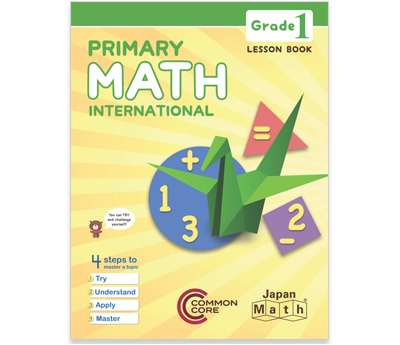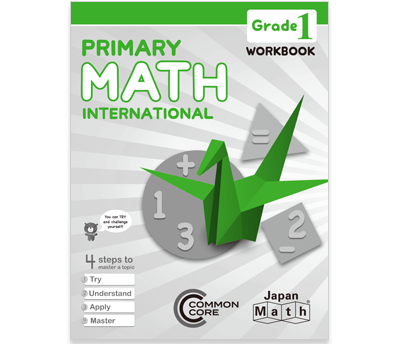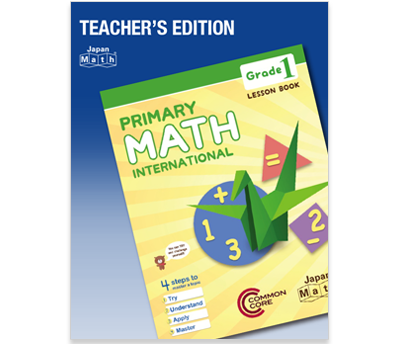# The Primary Math Curriculum

Our primary math program focuses on problem-solving and encourages students to find solutions on their own, with support and guidance from the teachers. We emphasize the development of a student’s ability to think for themselves and ensure they thoroughly understand fundamental concepts.

The goal of the primary math curriculum is to develop the student’s interest and skills to use math for life.

### The Concept: How Students Learn Through Problem-Solving

Students learn each topic through four steps: Try, Understand, Apply and Master. These steps reflect "learning through problem-solving" in actual classroom activities.

• Try: At the beginning of each lesson, students will try to solve a new problem themselves. Next, they discuss their ideas with the class and at the end of the lesson, return to their work to reflect on their findings. Trying familiarizes students with new topics.
• Understand: Students organize key concepts and procedures they have learned from the introductory problem. Then, they deepen their understanding by working on problems that follow the pattern of this he introductory problem. After exploring several examples, they summarize their understanding in their own words.
• Apply: Students solve problems with different variations, explain the procedures in their own words and share creative ways to solve problems with the class.
• Master: Students work on advanced problems that are more practical or that combine multiple concepts, then explain and evaluate solutions in small groups. Finally, they review the entire topic and summarize what they have learned.### What's Unique:  Integration of Lesson Book and Notebook

Each Japan Math program includes a lesson book that gives students writing space to record their calculations and thinking processes. Our lesson books serve as both the textbook and a notebook for students to record their thoughts, which they can use later on to help compound their understanding.

Our workbook pairs with the lesson book to give students a place to write down their own ideas and the ideas of other students. By verbally explaining ideas in their notes, students can check, correct and deepen their understanding of mathematical concepts. Each learning step provides a blank space for writing notes related to the topic and room for students to practice exercises.

Using Primary Math International, students will acquire not only knowledge of mathematics but also effective learning strategies.

### Product LineupLesson Book (Kindergarten - Grade 3)

The Lesson Book is provided for each student, and functions as a textbook and a notebook.